Next: Gaussian Probability Distribution Up: Probability Theory Previous: Mean, Variance, and Standard

# Application to Binomial Probability Distribution

Let us now apply what we have just learned about the mean, variance, and standard deviation of a general probability distribution function to the specific case of the binomial probability distribution. Recall, from Section 2.6, that if a simple system has just two possible outcomes, denoted 1 and 2, with respective probabilitiesand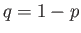, then the probability of obtaining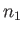occurrences of outcome 1 in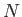observations is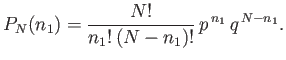(2.38)

Thus, making use of Equation (2.27), the mean number of occurrences of outcome 1 inobservations is given by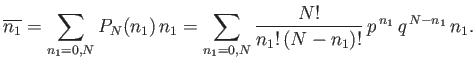(2.39)

We can see that if the final factorwere absent on the right-hand side of the previous expression then it would just reduce to the binomial expansion, which we know how to sum. [See Equation (2.23).] We can take advantage of this fact using a rather elegant mathematical sleight of hand. Observe that because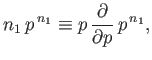(2.40)

the previous summation can be rewritten as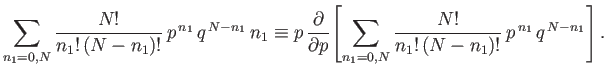(2.41)

The term in square brackets is now the familiar binomial expansion, and can be written more succinctly as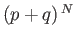. Thus,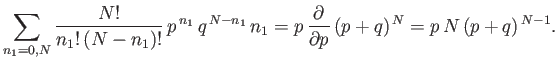(2.42)

However,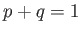for the case in hand [see Equation (2.11)], so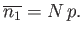(2.43)

In fact, we could have guessed the previous result. By definition, the probability,, is the number of occurrences of the outcome 1 divided by the number of trials, in the limit as the number of trials goes to infinity: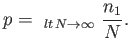(2.44)

If we think carefully, however, we can see that taking the limit as the number of trials goes to infinity is equivalent to taking the mean value, so that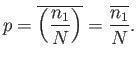(2.45)

But, this is just a simple rearrangement of Equation (2.43).

Let us now calculate the variance of. Recall, from Equation (2.36), that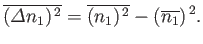(2.46)

We already know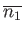, so we just need to calculate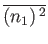. This average is written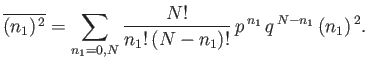(2.47)

The sum can be evaluated using a simple extension of the mathematical trick that we used previously to evaluate. Because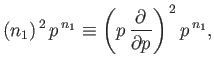(2.48)

then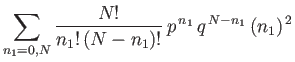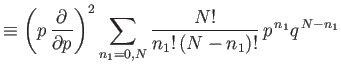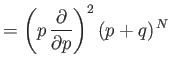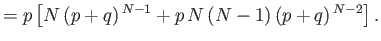(2.49)

Using, we obtain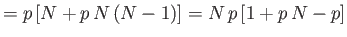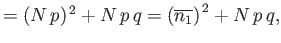(2.50)

because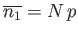. [See Equation (2.43).] It follows that the variance ofis given by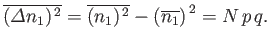(2.51)

The standard deviation ofis the square root of the variance [see Equation (2.37)], so that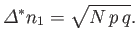(2.52)

Recall that this quantity is essentially the width of the range over whichis distributed around its mean value. The relative width of the distribution is characterized by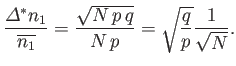(2.53)

It is clear, from this formula, that the relative width decreases with increasinglike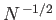. So, the greater the number of trials, the more likely it is that an observation ofwill yield a result that is relatively close to the mean value,.Next: Gaussian Probability Distribution Up: Probability Theory Previous: Mean, Variance, and Standard
Richard Fitzpatrick 2016-01-25